Centripetal Acceleration

Consider an object moving in a circle of radius r with constant angular velocity. The tangential speed is constant, but the direction of the tangential velocity vector changes as the object rotates.

Definition: Centripetal Acceleration

Centripetal acceleration is the rate of change of tangential velocity: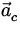=(17)

Note:

• The direction of the centripital acceleration is always inwards along the radius vector of the circular motion.
• The magnitude of the centripetal acceleration is related to the tangential speed and angular velocity as follows:

 ac =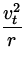=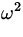r. (18)

• In general, a particle moving in a circle experiences both angular acceleration and centripetal accelaration. Since the two are always perpendicular, by definition, the magnitude of the net acceleration a total is:

 a total =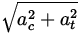=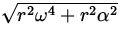. (19)

Definition: Centripetal Force

Centripetal force is the net force causing the centripetal acceleration of an object in circular motion. By Newton's Second Law: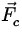= m. (20)

Its direction is always inward along the radius vector, and its magnitude is given by:

 Fc = mac = m= mr. (21)Next: Newton's Law of Gravitation Up: Circular Motion and the Previous: Relationship Between Linear and